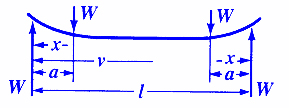Bending, Deflection and Stress Equations Calculator for

Beam Deflection and Stress Formula and Calculators

Structural Beam Deflection, Stress, Bending Equations and calculator for a Beam Supported on Both Ends Loaded Two equal Loads.Stress between nearest load and support pointStress at each load application and all points betweenDeflection between nearest load and support pointMaximum Deflection at CenterWhere:

 E = Modulus of Elasticity psi (N/mm2) I = Moment of Inertia in4 (mm4) W = Load on Beam lbs (N) s = Stress at the cross-section being evaluated Lbs/in2 (N/mm2) y = Deflection (Positive Upward Deflection, Negative Downward inches (mm) x = Some distance as indicated inches (mm) a = Some distance as indicated inches (mm) v = Some distance as indicated inches (mm)= Some distance as indicated inches (mm) Z = section modulus of the cross-section of the beam = I/z in3 (mm3) z = distance from neutral axis to extreme fiber (edge) inches (mm)
• Please note letter "" (lower case "L") is different than "I" (Moment of Inertia).
• Deflections apply only to constant cross sections along entire length.

References:

• Any Machinery's Handbook published since 1931 or,
• Machinery's Handbook, 21st Edition, Page 405 or,
• Machinery's Handbook, 23st Edition, Page 261 or,
• Machinery's Handbook, 27st Edition, Page 262Membership Register | LoginHomeEngineering Book StoreEngineering ForumExcel App. DownloadsOnline Books & ManualsEngineering NewsEngineering VideosEngineering CalculatorsEngineering ToolboxGD&T Training Geometric Dimensioning TolerancingDFM DFA TrainingTraining Online EngineeringAdvertising CenterCopyright Notice